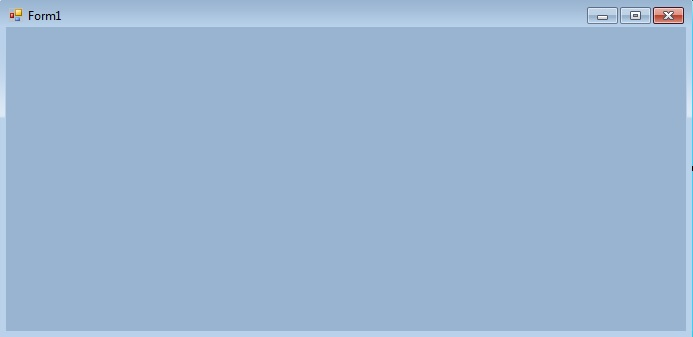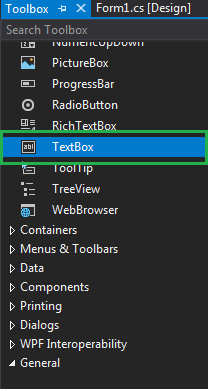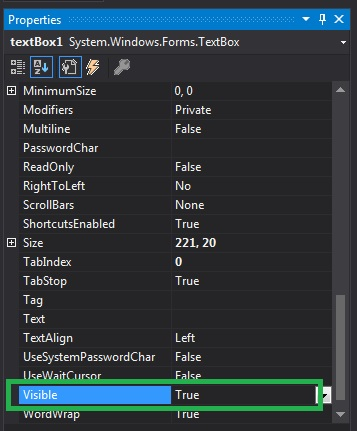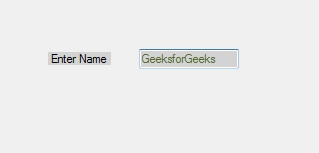Related Articles

# How to set the Visibility of the TextBox in C#?

• Last Updated : 29 Nov, 2019

In Windows forms, TextBox plays an important role. With the help of TextBox, the user can enter data in the application, it can be of a single line or of multiple lines. In TextBox, you are allowed to set a value which shows the TextBox control and its all child TextBox controls are displayed and it is achieved by using the Visible property of the TextBox. If you want to display the given TextBox and its child controls, then set the value of Visible property to true, otherwise set false. The default value of this property is true. In Windows form, you can set this property in two different ways:

1. Design-Time: It is the simplest way to set the Visible property of the TextBox as shown in the following steps:

• Step 1: Create a windows form as shown in the below image:
Visual Studio -> File -> New -> Project -> WindowsFormApp• Step 2: Drag the TextBox control from the ToolBox and drop it on the windows form. You can place TextBox anywhere on the windows form according to your need.• Step 3: After drag and drop you will go to the properties of the TextBox control to set the Visible property of the TextBox.Output:2. Run-Time: It is a little bit trickier than the above method. In this method, you can set the Visible property of the TextBox programmatically with the help of given syntax:

`public bool Visible { get; set; }`

Here, the value of this property is of System.Boolean type. Following steps are used to set the Visible property of the TextBox:

• Step 1 : Create a textbox using the TextBox() constructor provided by the TextBox class.
```// Creating textbox
TextBox Mytextbox = new TextBox();
```
• Step 2 : After creating TextBox, set the Visible property of the TextBox provided by the TextBox class.
```// Set Visible property
Mytextbox.Visible = true;
```
• Step 3 : And last add this textbox control to from using Add() method.
```// Add this textbox to form
 `using` `System;``using` `System.Collections.Generic;``using` `System.ComponentModel;``using` `System.Data;``using` `System.Drawing;``using` `System.Linq;``using` `System.Text;``using` `System.Threading.Tasks;``using` `System.Windows.Forms;`` ` `namespace` `my {`` ` `public` `partial` `class` `Form1 : Form {`` ` `    ``public` `Form1()``    ``{``        ``InitializeComponent();``    ``}`` ` `    ``private` `void` `Form1_Load(``object` `sender, EventArgs e)``    ``{`` ` `        ``// Creating and setting the properties of Lable1``        ``Label Mylablel = ``new` `Label();``        ``Mylablel.Location = ``new` `Point(96, 54);``        ``Mylablel.Text = ``"Enter Name"``;``        ``Mylablel.AutoSize = ``true``;``        ``Mylablel.BackColor = Color.LightGray;`` ` `        ``// Add this label to form``        ``this``.Controls.Add(Mylablel);`` ` `        ``// Creating and setting the properties of TextBox1``        ``TextBox Mytextbox = ``new` `TextBox();``        ``Mytextbox.Location = ``new` `Point(187, 51);``        ``Mytextbox.BackColor = Color.LightGray;``        ``Mytextbox.ForeColor = Color.DarkOliveGreen;``        ``Mytextbox.AutoSize = ``true``;``        ``Mytextbox.Name = ``"text_box1"``;``        ``Mytextbox.Visible = ``true``;`` ` `        ``// Add this textbox to form``        ``this``.Controls.Add(Mytextbox);``    ``}``}``}`